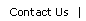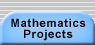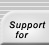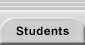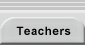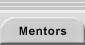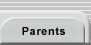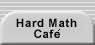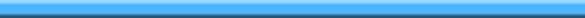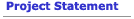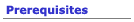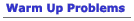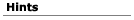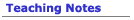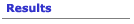## Hints for the Trains Problem

Here are two worries that people have when working on Trains:
• I’m not sure I have all the trains of length 5. People often miss trains when they’re just trying to build all the trains of length 5. If you’re not sure you found them all, try building all the trains of length 1, 2, 3, and 4. A pattern in the total number of trains should jump out at you. If you’re missing a few of the length 5 trains, knowing that they’re missing may help you figure out what they are.
• I have counted everything, but am not sure how to create an algorithm. Recursive algorithms are often the most successful ones for creating all the trains of a given length. The idea is, suppose you have a complete list of all the trains of length 4. Can you find a way to turn those into the trains of length 5? Look for some systematic way to do it. (Alternatively, suppose you have a complete list of all the trains of length 1, 2, 3, and 4. Can you use those to create the trains of length 5?)

Let’s look at a couple strategies for solving the Warm Up Problems. These may give you some ideas for Trains.

#### Algorithm 1: A Tree

Suppose you’re trying to build all the n-digit numbers that use only 1s and 2s. The idea is to start with one-digit numbers and build up from there. The one digit numbers are easy: 1 and 2.

To get the 2-digit numbers, you can either stick a 1 or a 2 onto the end of the existing one-digit numbers. To get the 3-digit numbers, you can either stick a 1 or a 2 onto the end of the existing two-digit numbers.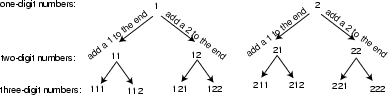And so on. You can move systematically down this tree. Each time you move to the left, add a 1 to the end of your number. Each time you move to the right, add a 2 to the end of your number.

Important things to prove: At any level in the tree, you have all the numbers you want (there aren’t any missing), and you don’t have any duplications. Also, how does this algorithm help you find the total number of n-digit numbers using only 1s and 2s?

#### Algorithm 2: Fill in the Gap

Suppose you successfully found all the (n - 1)-digit numbers that use only 1s and 2s. Now think about an n-digit number. It must start with either a 1 or a 2, and then it’s followed by an (1)-digit number. If you have a complete list of those, you’re done.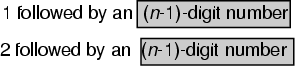Translations of mathematical formulas for web display were created by tex4ht. © Copyright 2003 Education Development Center, Inc. (EDC)